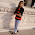## Saturday, December 17, 2011

### Comment on update to plotSimmap()

I just wanted to make a quick comment on my recent update to the phytools function plotSimmap() which now allows it to plot both "phylo" and "multiPhylo" SIMMAP style trees. As a preliminary, an object of class "multiPhylo" is just a list of "phylo" objects with class(...)<-"multiPhylo". To see that this is true, just make a list of "phylo" objects, set the class to "multiPhylo", and then apply any function that accepts "multiPhylo" objects to this list.

For instance, if we want to simulate 100 pure-birth trees we might want to use the geiger function birthdeath.tree. The only problem is it only simulates one tree at a time. To generate a set of (say) 100 pure-birth trees with 100 taxa, we can just do the following:

> require(geiger)
> trees<-replicate(100,birthdeath.tree(b=1,d=0,taxa.stop=100),simplify=F)
> "multiPhylo"->class(trees)
> plot.multiPhylo(trees) # we can also use the generic plot(...) here

Now, the way that plot.multiPhylo is simply by looping over each element in the "multiPhylo" object and calling the function plot.phylo(), after first setting the graphical parameter par(ask=TRUE) which will force R to ask the user for input (click or ENTER) before drawing each new tree. We can easily replicate this in plotSimmap() as follows:

plotSimmap<-function(tree,...){
if(class(tree)=="multiPhylo"){
for(i in 1:length(tree)) plotSimmap(tree[[i]],...)
} else { ...

If we do this, it works great! So for instance (after getting the new version of phytools here):

> require(geiger); require (phytools)
> tree<-rescaleTree(birthdeath.tree(b=1,d=0,taxa.stop=100),1)
> mtree<-sim.history(tree,Q=matrix(c(-1,1,1,-1),2,2))
> mtrees<-make.simmap(tree,mtree\$states,nsim=10)
> plotSimmap(mtrees)
Waiting to confirm page change...

Very cool.

1.2.